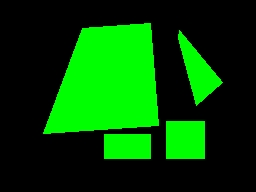# Replace Blob

The replace blob module will replace white blobs present in the current image with the specified color object shape as selected in the interface. The shapes' sizes will be based on the area of the blob being replaced. This module is useful if you want to better define the shape of the blob with a known shape. For example, replacing detected blobs with a better defined circle can aid in subsequent detection or analysis.

## Interface## Instructions

1. Color - Select the color the replaced shape will appear in. "mean" (last color) will cause the shape to be drawn in the average color of the original blob.

2. Shape - Select the shape that is used to replace each blob present in the image.

 bounding box - replaces each blob with it bounding box extents (xmax, ymax, xmin, ymin) minimal enclosure - replaces each blob with its minimal enclosure parallelogram (rectangle) circular enclosure - replaces each blob with a circle that encompasses the entire blob (aka Circumscribed Circle) inscribed circle - replaces each blob with the largest inner circle that fits into the blob crosshair - marks each blob's center of gravity with a cross hair square area - replaces each blob with a square of the same area centered at the center of gravity of the blob circle area - replaces each blob with a circle of the same area centered at the center of gravity of the blob triangle area - replaces each blob with a triangle of the same area centered at the center of gravity of the blob star area - replaces each blob with a star of the same area centered at the center of gravity of the blob diamond area - replaces each blob with a diamond of the same area centered at the center of gravity of the blob inner square - replaces each blob with the largest Cartesian (not rotated) square that fits in the blob inner rectangle - replaces each blob with the largest Cartesian (not rotated) rectangle that fits in the blob minimal quadrilateral - replaces each blob with the largest 4 sides polygon that fits around the blob minimal parallelogram - replaces each blob with the largest parallelogram that fits around the blob ellipse - replaces each blob with an ellipse best fit rectangle - uses a best fit algorithm to create 4 lines that best represent all the edge points of the blob

3. Thickness - If the shape is drawn as lines this specifies how thick the drawn line should be.

4. Fill Shape - Select if you want the object shape to be filled in.

5. Overlay - Select what the resulting graphic will be drawn on.

Source - the original captured image
Current - the current image seen at this point in the processing pipeline
XXX - other images created by the Marker module.

6. Order - Each replace creates variables (see below) that contain the values that represent the replaced blob. The order of these blobs and thus their numerical results can be changed by selecting the order checkboxes. For example, if you want to order the array in increasing X then select the "Order array based on X" which will sort the blobs in increasing X order. This can be handy if you are relying on a specific order of the blobs in order to continue processing in subsequent modules or scripts.

## Example

 Source - Minimal Quadrilateral-The example above shows an image RGBFiltered, Dilated and then blob replaced with red circles. Notice how the red traffic light is now better pronounced than in the source image.

## Variables

```BLOB_REPLACE_COORDINATES - duplicate array from one of the following arrays. Can be used
to avoid having to rename array references when switching between different replace
methods.

BOUNDING_COORDINATES - p1x, p1y, p2x, p2y, p3x, p3y, p4x, p4y coordinates of the Bounding
Box of the replaced blob when this is selected. Note that this is an ARRAY with
8 numbers per blob.

CROSSHAIR_COORDINATES - x, y coordinates of the CrossHair that replaces a blob
when this replace method is selected. Note that this is an ARRAY with 2 numbers
per replaced blob.

SQUARE_COORDINATES - p1x, p1y, p2x, p2y, p3x, p3y, p4x, p4y  coordinates of the Square
that replaces the blob when this is selected. Note that this is an ARRAY with
8 numbers per blob.

CIRCLE_COORDINATES - x, y, radius of the Circle that replaces the blob when this is
selected. Note that this is an ARRAY with 3 numbers per blob.

TRIANGLE_COORDINATES - x, y, base of the Triangle that replaces the blob when this is
selected. Note that this is an ARRAY with 3 numbers per blob.

STAR_COORDINATES - x, y, radius of the Star that replaces the blob when this is
selected. Note that this is an ARRAY with 3 numbers per blob.

DIAMOND_COORDINATES - x, y, radius of the Diamond that replaces the blob when this is
selected. Note that this is an ARRAY with 3 numbers per blob.

BFR_COORDINATES - p1x, p1y, p2x, p2y, p3x, p3y, p4x, p4y coordinates of the Best Fit
Rectangle of the replaced blob when this is selected. Note that this is an ARRAY with
8 numbers per blob.

MEQ_COORDINATES - p1x, p1y, p2x, p2y, p3x, p3y, p4x, p4y coordinates of the Minimal
Quadrilateral of the replaced blob when this is selected. Note that this is an ARRAY with
8 numbers per blob.

MER_COORINATES - p1x, p1y, p2x, p2y, p3x, p3y, p4x, p4y coordinates of the Minimal Enclosed
Rectangle for each detected blob when the . Note that this is an ARRAY with 8 numbers per blob.

MEP_COORINATES - p1x, p1y, p2x, p2y, p3x, p3y, p4x, p4y coordinates of the Minimal Enclosed
Polygon for each detected blob. Note that this is an ARRAY with 8 numbers per blob.
```